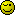# Calendar Days Of Week Error

Hi All, I’ve grabbed a php calendar script which runs sun to sat, I need it to run mon - sun but cant seem to get the changes right for it to work. Anyone give me some help. Many thanks

<tr>
<td width=“265” valign=“top”><?php
if (isset(\$_GET[‘day’])){
\$day = \$_GET[‘day’];
} else {
\$day = date(“j”);
}
if(isset(\$_GET[‘month’])){
\$month = \$_GET[‘month’];
} else {
\$month = date(“n”);
}
if(isset(\$_GET[‘year’])){
\$year = \$_GET[‘year’];
}else{
\$year = date(“Y”);
}
\$currentTimeStamp = strtotime( “\$day-\$month-\$year”);
\$monthName = date(“F”, \$currentTimeStamp);
\$numDays = date(“t”, \$currentTimeStamp);
\$counter = 0;
?>
<br />
<table border=‘1’>
<tr>
<td><input style=‘width:50px;’ type=‘button’ value=‘<‘name=‘previousbutton’ onclick =“goLastMonth(<?php echo \$month.”,“.\$year?>)”></td>
<td colspan=‘5’><?php echo \$monthName.", ".\$year; ?></td>
<td><input style=‘width:50px;’ type=‘button’ value=’>‘name=‘nextbutton’ onclick =“goNextMonth(<?php echo \$month.”,“.\$year?>)”></td>
</tr>
<tr>
<td width=‘25px’>Mon</td>
<td width=‘25px’>Tue</td>
<td width=‘25px’>Wed</td>
<td width=‘25px’>Thu</td>
<td width=‘25px’>Fri</td>
<td width=‘25px’>Sat</td>
<td width=‘25px’>Sun</td>
</tr>
<?php
echo “<tr>”;
for(\$i = 1; \$i < \$numDays+1; \$i++, \$counter++){
\$timeStamp = strtotime(“\$year-\$month-\$i”);
if(\$i == 1) {
\$firstDay = date(“w”, \$timeStamp);
for(\$j = 1; \$j < \$firstDay; \$j++, \$counter++) {
echo “<td> </td>”;
}
}
if(\$counter % 7 == 0) {
echo"</tr><tr>";
}
\$monthstring = \$month;
\$monthlength = strlen(\$monthstring);
\$daystring = \$i;
\$daylength = strlen(\$daystring);
if(\$monthlength <= 1){
\$monthstring = “0”.\$monthstring;
}
if(\$daylength <=1){
\$daystring = “0”.\$daystring;
}
\$todaysDate = date(“m/d/Y”);
\$dateToCompare = \$monthstring. ‘/’ . \$daystring. ‘/’ . \$year;
echo "<td align=‘center’ ";
if (\$todaysDate == \$dateToCompare){
echo “class =‘today’”;
}
echo "><a href=’“.\$_SERVER[‘PHP_SELF’].”?month=“.\$monthstring.”&day=“.\$daystring.”&year=“.\$year.”&v=true’>“.\$i.”</a></td>";
}
echo “</tr>”;
?>
</table>

That’s… cluttered. But i suppose it works.
Basically, the answer is “add 6 to the weekday of the first date of the month, and modulo it by 7”.

See if you can figure out where that goes.Hi thanks for the tip, Im really stumped, it all works fine until 1st July which is falling on a Monday and not Sunday which it should be. I’m guessing the answer is in
for(\$j = 1; \$j < \$firstDay; \$j++, \$counter++)
{
echo “<td> </td>”;
}

but nothing works!

Almost. That loop uses the first day of the month, but it isnt the line that’s deciding what weekday the first day of the month is.

fixed that will teach me to modify someones code and not write from scratch

@SteveWhea

The script you supplied would be a lot better wrapped in PHP tags.

I tried your script locally and the calendar displays correctly starting at Sunday but the missing script did not allow the month to be changed.

Perhaps Google for another script and with a bit of luck may take less time to install than to find the errors in the existing script.

Hi

I downloaded and modified it several years ago on another project, so made a few adjustments on the current version as needed days of week Mon - Sun.

<script>
function goLastMonth(month, year){
if(month == 1) {
–year;
month = 13;
}
–month
var monthstring= “”+month+“”;
var monthlength = monthstring.length;
if(monthlength <=1){
monthstring = “0” + monthstring;
}
document.location.href =“<?php \$_SERVER[‘PHP_SELF’];?>?month=”+monthstring+“&year=”+year+“&v=true”;
}
function goNextMonth(month, year){
if(month == 12) {
++year;
month = 0;
}
++month
var monthstring= “”+month+“”;
var monthlength = monthstring.length;
if(monthlength <=1){
monthstring = “0” + monthstring;
}
document.location.href =“<?php \$_SERVER[‘PHP_SELF’];?>?month=”+monthstring+“&year=”+year+“&v=true”;
}
</script>
<style>
.today{
background-color: #00ff00;
}
.event{
background-color: #FF8080;
}
</style>

if (isset(\$_GET[‘day’])){
\$day = \$_GET[‘day’];
} else {
\$day = date(“j”);
}
if(isset(\$_GET[‘month’])){
\$month = \$_GET[‘month’];
} else {
\$month = date(“n”);
}
if(isset(\$_GET[‘year’])){
\$year = \$_GET[‘year’];
}else{
\$year = date(“Y”);
}
\$currentTimeStamp = strtotime( “\$day-\$month-\$year”);
\$monthName = date(“F”, \$currentTimeStamp);
\$numDays = date(“t”, \$currentTimeStamp);
\$counter = 0;
?>
<br />
<table border=‘1’>
<tr>
<td><input style=‘width:50px;’ type=‘button’ value='<‘name=‘previousbutton’ onclick =“goLastMonth(<?php echo \$month.”,“.\$year?>)”></td>
<td colspan=‘5’><?php echo \$monthName.", ".\$year; ?></td>
<td><input style=‘width:50px;’ type=‘button’ value=’>'name=‘nextbutton’ onclick =“goNextMonth(<?php echo \$month.”,“.\$year?>)”></td>
</tr>
<tr>
<td width=‘25px’>Mon</td>
<td width=‘25px’>Tue</td>
<td width=‘25px’>Wed</td>
<td width=‘25px’>Thu</td>
<td width=‘25px’>Fri</td>
<td width=‘25px’>Sat</td>
<td width=‘25px’>Sun</td>
</tr>
<?php
echo “<tr>”;
for(\$i = 1; \$i < \$numDays+1; \$i++, \$counter++)
{
\$timeStamp = strtotime(“\$year-\$month-\$i”);
if(\$i == 1)
{
\$firstDay = date(“w”, \$timeStamp);

``````				if(\$firstDay==0)
{
for(\$j = 1; \$j &lt; 7; \$j++, \$counter++)
{
echo "&lt;td&gt;&nbsp;&lt;/td&gt;";
}
}
else
{
for(\$j = 1; \$j &lt; \$firstDay; \$j++, \$counter++)
{
echo "&lt;td&gt;&nbsp;&lt;/td&gt;";
}
}
}
``````

if(\$counter % 7 == 0)
{
echo"</tr><tr>";
}

\$monthstring = \$month;
\$monthlength = strlen(\$monthstring);
\$daystring = \$i;
\$daylength = strlen(\$daystring);
if(\$monthlength <= 1){
\$monthstring = “0”.\$monthstring;
}
if(\$daylength <=1){
\$daystring = “0”.\$daystring;
}
\$todaysDate = date(“m/d/Y”);
\$dateToCompare = \$monthstring. ‘/’ . \$daystring. ‘/’ . \$year;
echo “<td align=‘center’ “;
if (\$todaysDate == \$dateToCompare){
echo “class =‘today’”;
}
echo “><a href='”.\$_SERVER[‘PHP_SELF’].”?month=”.\$monthstring.“&day=”.\$daystring.“&year=”.\$year.“&v=true’>”.\$i.“</a></td>”;
}
echo “</tr>”;
?>
</table>

Many thanks for the script, I will try it tomorrow.

Please take the time to learn how to wrap your script in code tags - left square bracket php right square bracket.

I use CodeIgniter which has a very flexible Calendar library, so much easier to implement and adjust.

http://codeigniter.com/user_guide/libraries/calendar.html

``````